Next:8.4.2 Optimal Solution for TSP using Branch and BoundUp:8.4 Traveling Salesman ProblemPrevious:8.4 Traveling Salesman Problem

## 8.4.1 A Greedy Algorithm for TSP

• Based on Kruskal's algorithm. It only gives a suboptimal solution in general.
• Works for complete graphs. May not work for a graph that is not complete.
• As in Kruskal's algorithm, first sort the edges in the increasing order of weights.
• Starting with the least cost edge, look at the edges one by one and select an edge only if the edge, together with already selected edges,
• 1.
does not cause a vertex to have degree three or more
2.
does not form a cycle, unless the number of selected edges equals the number of vertices in the graph.
Example:

Consider the six city problem shown in Figure 8.14. The sorted set of edges is

{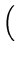(d, e), 3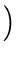,(b, c), 5,(a, b), 5,(e, f ), 5,(a, c), 7.08,(d, f ),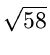,(b, e),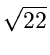,(b, d ),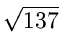,(c, d ), 14,...(a, f ), 18See Figures 8.15 and 8.16.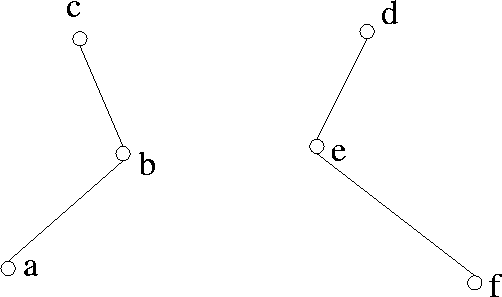Select (d, e) Select (a, b) Select (b, c) Select (e, f) Reject (a, c) since it forms a cycle with (a, b) and (b, c) Reject (d, f) since it forms a cycle with (d, e) and (e, f) Reject (b, e) since that would make the degree of b equal to 3 Reject (b, d) for an identical reason Select (c, d) . . . Select (a, f)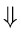This yields a total cost = 50, which is about 4% from the optimal cost.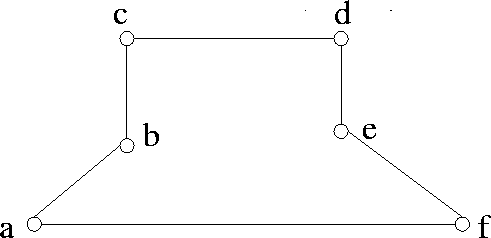Next:8.4.2 Optimal Solution for TSP using Branch and BoundUp:8.4 Traveling Salesman ProblemPrevious:8.4 Traveling Salesman Problem
eEL,CSA_Dept,IISc,Bangalore